### Home > A2C > Chapter 5 > Lesson 5.1.3 > Problem5-39

5-39.
1. This is a Checkpoint for use of function notation and describing domains and ranges.Find the domain and range of g(x) = 2(x + 3)2. Then answer the questions below. Homework Help ✎1. Find g(−5).

2. Find g(a + 1).

3. If g(x) = 32, figure out what number x can be.

4. If g(x) = 0, figure out what number x can be.

5. Check your answers by referring to the Checkpoint 9 materials located at the back of your book.

If you needed help to solve these problems correctly, then you need more practice using function notation and describing domains and ranges. Review the Checkpoint 9 materials and try the practice problems. Also, consider getting help outside of class time. From this point on, you will be expected to solve problems like these easily and accurately.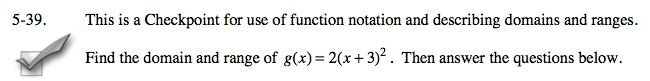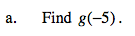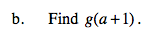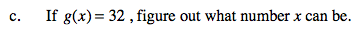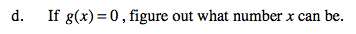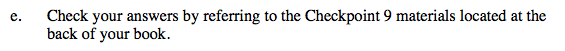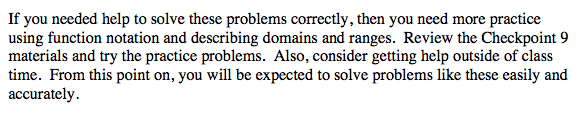Answers and extra practice are located in the back of your printed textbook or in the Reference Tab of your eBook.
If you have an eBook for A2C , login and then click the following link: Checkpoint 9 Answers and Practice